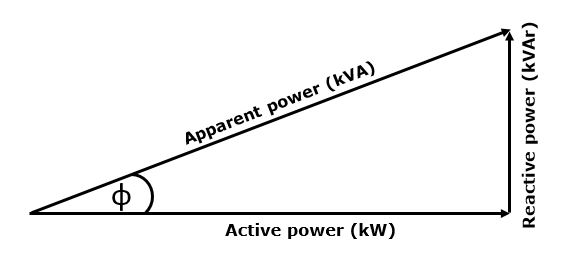# Reduce Your Electricity Costs with Power Factor Correction

Demand charges are based on the peak apparent power supplied to electrical energy users, measured in kilovolt-amperes (kVA). Load shifting, where individual loads are scheduled such that the overall peak demand on a site is reduced, is a viable strategy for reducing demand charges. It can be achieved through active management of individual loads or by using a demand controller. Energy efficiency projects that reduce input power (remember that energy efficiency can also be achieved by reducing running time) will reduce active power consumption, and hence also reduce demand. These two approaches can be combined with a third strategy – that of power factor correction. This strategy requires some investment, but is a simple, proven method for reducing demand and also, to a lesser extent, energy consumption. While in many cases implementing power factor correction can be considered to be a “no-brainer”, I still come across sites where it is not in place, systems are insufficient, or systems are not functional. So what is power factor correction, and why should you be looking at it seriously if you don’t have an effectively functioning system?

There are three types of loads to be considered on industrial and commercial sites: resistive loads, inductive loads and capacitive loads. The quantum and mix of these loads determines the overall power factor of a site, and hence the degree of correction required.

Resistive loads draw current with voltage and current in phase. Resistive loads include electrical heaters and loads such as (increasingly uncommon) incandescent lights. You will find them in machines such as shrink-wrappers, electrically heated process vessels and heat tracing systems for pipelines, as examples.

Inductive and capacitive loads draw current with voltage and current out of phase, with voltage leading the current for inductive loads and current leading the voltage for capacitive loads.  In general, capacitive loads do not make up a significant portion of an industrial site’s demand. Inductive loads are therefore the primary concern as regards power factor correction.

Inductive loads are found in devices that make use of electromagnetic fields to operate, such as motors, transformers, induction furnaces and the ballasts for some types of lighting systems. They tend to be the dominant drivers of an industrial site’s demand. Capacitive loads can be introduced to offset inductive loads, and this is the principle behind the design of a power factor correction system.

The power factor of a load is the ratio of the active power supplied to the load to the apparent power supplied to the load. Apparent power is the product of voltage and current, and is the vector sum of active and reactive power. Purely resistive loads have a power factor of unity, meaning that active and apparent power are equal. Inductive loads have a power factor that is less than unity due to their reactive power component. Apparent power, active power and reactive power are related to each other through the “power triangle”, as shown below.The impedance phase angle, φ, is the angle by which the current and voltage are out of phase.

Power factor = Active Power / Apparent power = cos φ

Power factor correction seeks to reduce or eliminate reactive power due to inductive loads, reducing or eliminating the phase angle, and moving apparent power closer to active power. This in turn reduces maximum demand. To achieve this, capacitors are used to offset the inductive reactive power, with the amount of capacitance needed determined by the quantum of the reactive power involved. The power factor correction system will also be equipped with a controller to control the switching of the capacitors, and possibly a filter should harmonic distortion be a concern.

Power factor correction can be quite an abstract concept, so let me illustrate its impact via a practical example. The graph below is an extract from an actual logging exercise carried out on a power supply to a small manufacturing site.The red line, which represents apparent power, contains two peaks, suggesting a maximum demand of roughly 500 kVA. Active power consumption peaks (at the same two corresponding points) at around 475 kW. The power factor at maximum demand is therefore PF = 475/500 = 0.95. This particular site already had a power factor correction system in place, and improving the power factor was then a case of augmenting the installed capacitance, rather than the installation of a new system. The maximum demand tariff for this site was R160/kVA. The demand benefits of correcting the power factor to unity would be 25kVA/month, or 300kVA/annum, which yields a saving of R48,000/annum. The reactive power to be offset would be 156 kVAr, at a cost for the additional capacitance of R32,225. The payback for this intervention would only be 0.65 years. For this site, augmentation of the existing system is therefore attractive. Implementation would cause the red line in the graph to migrate closer to the blue line.